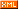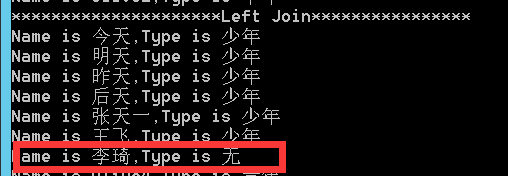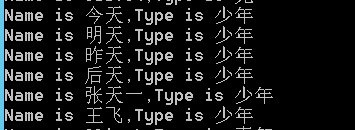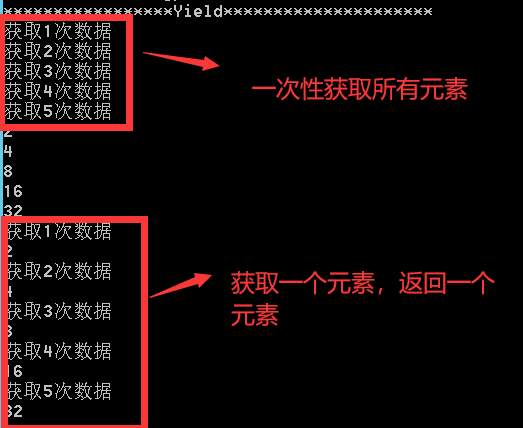# 星星之火

posts - 127, comments - 374, trackbacks - 0, articles - 3
::  ::  ::  ::::

# 1. Linq

Linq 其实就是把对数据操作的通用部分完成，把可变的交给委托，使用者只用关心可变部分，其实Linq就是这么一个封装。

## 1.1        Linq常用方法介绍

### 1.1.1  Where

List<Person> list = new List<Person>()

{

new Person(){ Id=1,Name="今天",Age=10,TypeId=1},

new Person(){ Id=2,Name="明天",Age=15,TypeId=1},

new Person(){ Id=3,Name="昨天",Age=20,TypeId=1},

new Person(){ Id=4,Name="后天",Age=25,TypeId=1},

new Person(){ Id=5,Name="张天一",Age=10,TypeId=1},

new Person(){ Id=6,Name="王飞",Age=15,TypeId=1},

new Person(){ Id=7,Name="李琦",Age=20}};

var result = list.Where<Person>(item => item.Age < 15);

### 1.1.2  Select

var result=list.Select(item => new { Name = item.Name, Age = item.Age })//投影

### 1.1.3  Join

List<PType> pTypeList = new List<PType>()

{

new  PType(){ Id=1,Name="少年"},

new  PType(){ Id=2,Name="青年"},

new  PType(){ Id=3,Name="中年"}

};

var reuslt = from p in list

join pt in pTypeList on p.TypeId equals pt.Id

select new

{

Name = p.Name,

Type = pt.Name

};

var result1 = list.Join(pTypeList, p => p.TypeId, pt => pt.Id, (p, pt) => new

{

Name = p.Name,

Type = pt.Name

});

var result0 = from p in list

join pt in pTypeList on p.TypeId equals pt.Id

into pptList

from ppt in pptList.DefaultIfEmpty()

select new

{

Name =p.Name,

Type = (ppt!=null?ppt.Name:"无")

};var result1 = list.Join(pTypeList, p => p.TypeId, pt => pt.Id, (p, pt) => new

{

Name = p.Name,

Type = pt.Name

}).DefaultIfEmpty();//这种形式如果关联不上，则该条数据不存在### 1.1.4  Group

var gList = list.GroupBy(item => item.Age)//按年龄分组

.Select(item => new { Age = item.Key, Count = item.Count() });//求个年龄人数

### 1.1.5  OrderBy

var result=list.OrderBy(item => item.Age)//升序排列

.OrderByDescending(item => item.Age)//降序排列

.Skip(1)//跳过几条

.Take(5);//取几条

## 1.3        Yield迭代器

public IEnumerable<int> CommonMethod()

{

List<int> result = new List<int>();

int counter = 0;

int r = 1;

while(counter++<5)

{

Console.WriteLine($"获取{counter}次数据"); r = r * 2; result.Add(r); } return result; } Yield方法： public IEnumerable<int> YieldMethod() { int counter = 0; int r = 1; while(counter++<5) { Thread.Sleep(1000); Console.WriteLine($"获取{counter}次数据");